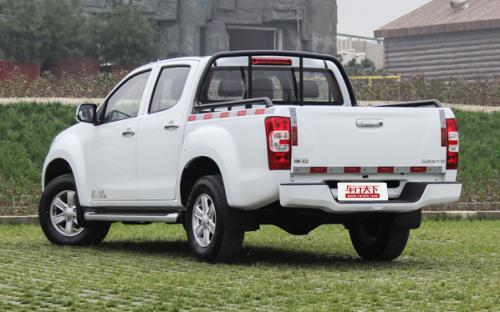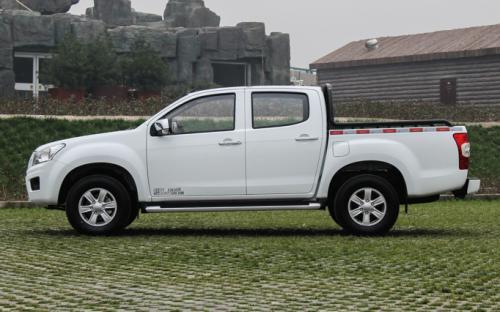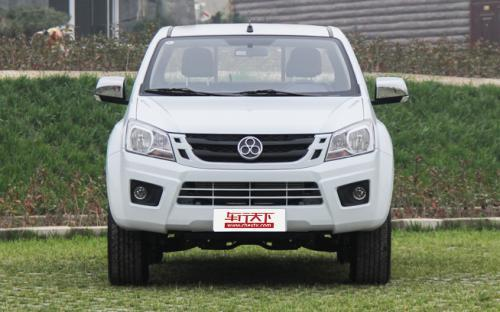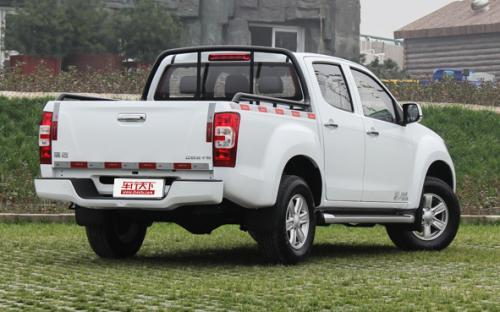### 江西五十铃 瑞迈0 种颜色可选2016款最低售价：8.48 万元起

5190×1860×1785(mm)(mm)(mm)##### 配置亮点：
• 胎压监测装置

• ISOFIX儿童座椅接口

• 车身稳定控制(ESC/ESP/DSC等)

• 电动天窗

• 定速巡航

• 后倒车雷达

• 真皮座椅

• GPS导航系统

• 氙气大灯

• 后视镜加热

• 提交
2016款 2.8T两驱豪华款JE493ZLQ4CA (96张)
• 2016款 2.8T两驱豪华款JE493ZLQ4CA (96张)
• 2016款 2.8T两驱超豪华款JE493ZLQ4CA (88张)
• 江西五十铃 瑞迈 绕车实拍• 江西五十铃 瑞迈 在售车型

排量 车型 厂商指导价 本地最低报价 购车工具
2.8L
2.8T两驱进取款JE493ZLQ4CA 柴油 5挡手动
8.48万
2.8T两驱豪华款JE493ZLQ4CA 柴油 5挡手动
8.88万
2.8T两驱精英款JE493ZLQ4CA 柴油 5挡手动
9.18万
9.88万
2.8T四驱豪华款JE493ZLQ4CA 柴油 5挡手动
10.68万
11.68万

江西五十铃 瑞迈 经销商

查看更多 >>

### 江西五十铃 瑞迈 动力加速

瑞迈 0-100公里加速时间分布在 0.0-秒 属于 超跑级

动力级别 加速时间 车型

江西五十铃 瑞迈 视频

江西五十铃 瑞迈 新闻资讯

# 江西五十铃瑞迈汽油版强势掀起订单热潮

行业动态 超过8992次关注

沐浴政策春风，柴油版瑞迈凭借强大的实用优势，一路高唱凯歌，连续刷新多个销售记录。而今，在汽油皮卡市场急需动能改造，排放升级的大环境下，汽油版瑞迈破世而出。

# 芯虔志承 瑞迈皮卡成就新春第一股订单热潮

新闻 超过9131次关注

瑞迈作为皮卡界的新锐，凭借五十铃在发动机、车身、底盘、装载以及性价比上的多重优势，继续沿续去年高歌猛进的势头，在行业内再起风云，掀起了新春第一股订单热潮。

# 江西五十铃瑞迈 瑞力勃发势不可挡

新闻 超过9575次关注

2017年是皮卡解禁政策试行的第二年，为了积极响应国家排放政策，也为了满足更多用户的需求，瑞迈将推出汽油版，在保持柴油市场涨势的同时，将在实用皮卡领域形成全...

# 瑞迈汽油低油耗与高动力挑T性能 一路芯动预售

评测 超过7546次关注

为了庆祝瑞迈皮卡在2016的辉煌表现、迎接汽油版瑞迈的芯动预售，瑞迈全系现推出全系钜惠。从2017年1月1日起，在活动期间消费者只要到店购买全系任何车型都将获得价...

# 瑞迈加长货箱上市 强力冲击轻卡及微面市场

行业动态 超过9584次关注

在今年的8月皮卡市场销量当中，整体销售数据迅速攀升，其中江西五十铃瑞迈车系的销量更是让行业看到皮卡的未来，随着瑞迈加长货箱的重磅上市，皮卡市场的销量有望取...

# 百年匠心厚载未来 江西五十铃瑞迈加长货箱预售开启

汽车导购 超过8784次关注

不知什么时候起，国内的汽车销售开始流行“加长版”，加个“L”以表明自己是加长版的身份。然而实际上，真正的加长版，远不是简单加个轴距那么简单，更要和车辆定位...

# 江西五十铃瑞迈的精品之道

行业动态 超过9172次关注

五十铃对于汽车爱好者来说是一个耳熟能详的品牌，作为国内生产该品牌汽车的江西五十铃一直也是在不断地探索、开拓新产品，瑞迈成为江西五十铃发展进程中不可忽视的...

猜你喜欢

﻿
• 快速找车
• 选择品牌
• 选择品牌
• A  奥迪
• A  阿斯顿·马丁
• A  阿尔法·罗密欧
• B  宝沃
• B  布加迪
• B  巴博斯
• B  保时捷
• B  宾利
• B  奔驰
• B  宝马
• B  本田
• B  别克
• B  标致
• B  比亚迪
• B  宝骏
• B  北汽制造
• B  北汽新能源
• B  北汽幻速
• B  北汽威旺
• B  北京汽车
• B  奔腾
• B  北汽绅宝
• C  长安
• C  长安商用
• C  长城
• C  昌河
• D  大众
• D  道奇
• D  DS
• D  东南
• D  东风风神
• D  东风风行
• D  东风小康
• D  东风风度
• D  东风
• F  福特
• F  丰田
• F  菲亚特
• F  法拉利
• F  福田
• F  福迪
• F  福汽启腾
• G  观致
• G  广汽传祺
• G  广汽吉奥
• G  GMC
• H  红旗
• H  汉腾汽车
• H  哈弗
• H  哈飞
• H  海格
• H  海马
• H  华颂
• H  黄海
• H  华泰
• H  恒天
• J  吉利汽车
• J  捷豹
• J  Jeep
• J  江淮
• J  江铃
• J  金杯
• J  九龙
• J  金旅
• K  凯翼
• K  凯迪拉克
• K  克莱斯勒
• K  科尼塞克
• K  卡威
• K  开瑞
• L  路虎
• L  林肯
• L  劳斯莱斯
• L  兰博基尼
• L  雷克萨斯
• L  铃木
• L  雷诺
• L  理念
• L  力帆
• L  莲花汽车
• L  猎豹
• L  路特斯
• L  陆风
• M  马自达
• M  MG
• M  MINI
• M  玛莎拉蒂
• M  摩根
• M  迈凯轮
• N  纳智捷
• O  欧宝
• O  讴歌
• O  欧朗
• Q  奇瑞
• Q  起亚
• Q  启辰
• R  日产
• R  荣威
• R  瑞麒
• S  三菱
• S  斯威汽车
• S  萨博
• S  smart
• S  斯柯达
• S  斯巴鲁
• S  思铭
• S  双龙
• S  上汽大通
• S  双环
• T  特斯拉
• T  腾势
• W  沃尔沃
• W  五菱汽车
• W  五十铃
• W  威兹曼
• W  威麟
• X  现代
• X  雪佛兰
• X  雪铁龙
• X  西雅特
• Y  一汽
• Y  英菲尼迪
• Y  英致
• Y  依维柯
• Y  野马汽车
• Y  永源
• Z  众泰
• Z  中华
• Z  中兴
• Z  知豆
• 选择车系
• 选择车系
• 车型对比
• 选择品牌
• 选择品牌
• A  奥迪
• A  阿斯顿·马丁
• A  阿尔法·罗密欧
• B  宝沃
• B  布加迪
• B  巴博斯
• B  保时捷
• B  宾利
• B  奔驰
• B  宝马
• B  本田
• B  别克
• B  标致
• B  比亚迪
• B  宝骏
• B  北汽制造
• B  北汽新能源
• B  北汽幻速
• B  北汽威旺
• B  北京汽车
• B  奔腾
• B  北汽绅宝
• C  长安
• C  长安商用
• C  长城
• C  昌河
• D  大众
• D  道奇
• D  DS
• D  东南
• D  东风风神
• D  东风风行
• D  东风小康
• D  东风风度
• D  东风
• F  福特
• F  丰田
• F  菲亚特
• F  法拉利
• F  福田
• F  福迪
• F  福汽启腾
• G  观致
• G  广汽传祺
• G  广汽吉奥
• G  GMC
• H  红旗
• H  汉腾汽车
• H  哈弗
• H  哈飞
• H  海格
• H  海马
• H  华颂
• H  黄海
• H  华泰
• H  恒天
• J  吉利汽车
• J  捷豹
• J  Jeep
• J  江淮
• J  江铃
• J  金杯
• J  九龙
• J  金旅
• K  凯翼
• K  凯迪拉克
• K  克莱斯勒
• K  科尼塞克
• K  卡威
• K  开瑞
• L  路虎
• L  林肯
• L  劳斯莱斯
• L  兰博基尼
• L  雷克萨斯
• L  铃木
• L  雷诺
• L  理念
• L  力帆
• L  莲花汽车
• L  猎豹
• L  路特斯
• L  陆风
• M  马自达
• M  MG
• M  MINI
• M  玛莎拉蒂
• M  摩根
• M  迈凯轮
• N  纳智捷
• O  欧宝
• O  讴歌
• O  欧朗
• Q  奇瑞
• Q  起亚
• Q  启辰
• R  日产
• R  荣威
• R  瑞麒
• S  三菱
• S  斯威汽车
• S  萨博
• S  smart
• S  斯柯达
• S  斯巴鲁
• S  思铭
• S  双龙
• S  上汽大通
• S  双环
• T  特斯拉
• T  腾势
• W  沃尔沃
• W  五菱汽车
• W  五十铃
• W  威兹曼
• W  威麟
• X  现代
• X  雪佛兰
• X  雪铁龙
• X  西雅特
• Y  一汽
• Y  英菲尼迪
• Y  英致
• Y  依维柯
• Y  野马汽车
• Y  永源
• Z  众泰
• Z  中华
• Z  中兴
• Z  知豆
• 选择车系
• 选择车系
• 选择车型
• 选择车型
• 意见反馈